# Speed Torque Characteristics of Series Motor

## Speed Torque Characteristics of Series Motor:

The field winding is connected in series with the armature (Fig. 1.2(b)). The armature current and field current are the same. The operating Speed Torque Characteristics of Series Motor may be deduced for a series motor in the same way as described before for shunt motor/separately excited motor using the basic equations (Eqs 1.3 and 1.4). The speed and armature current are related by the equation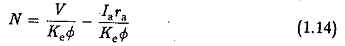whereas Td and la are related by

In a series motor If = la and hence the field flux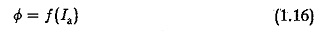For low values of armature current causing no saturation we have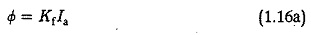using which we have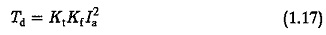substituting for Ia in Eq. 1.14, we have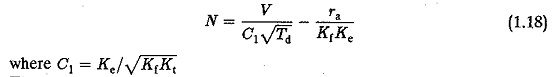The speed-torque characteristic of an unsaturated series motor is represented by AB in Fig. 1.10. As the torque increases, the speed drops rapidly to B.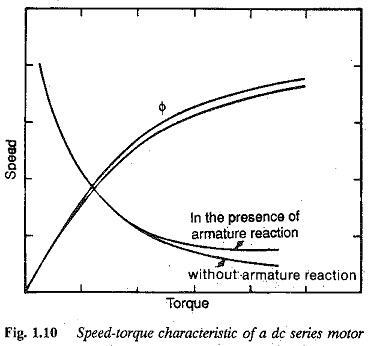When the armature current is greater than the value corresponding to point B, saturation sets in and the actual value of flux must be substituted in the equation to get the characteristic. However, to understand the trend of the curve it can be assumed that Φ is constant. Under this assumption the speed-torque characteristic of saturated series motor is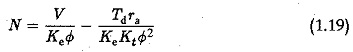and BC in Fig. 1.10 represents the characteristic. AC therefore, is a typical  speed-torque curve of a dc series motor. Also, the effect of armature reaction is predominant only when the armature current is large. The speed drop is restricted due to a decrease in the field flux. The effect of armature reaction is also depicted in Fig. 1.10.

### Effect of variation of armature voltage

The armature voltage variation to a series motor can be achieved by means of an additional resistance in the armature circuit or by using a thyristor power converter. On doing this the torque-speed curve moves towards the right when the voltage is increased. For a given torque developed the motor speed increases with an increase in voltage. The speed-torque curves for different armature voltages (for different armature resistances) are shown in Fig. 1.11. Of the two methods employed for the variation of the voltage the use of additional resistance has greater losses, leading to poor efficiency.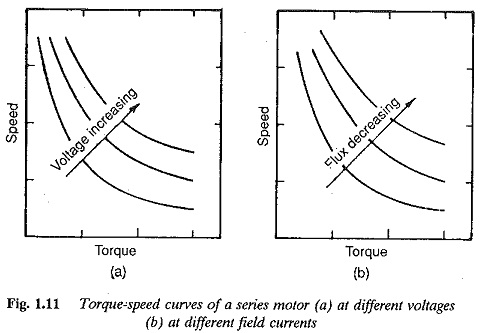### Field weakening in series motor

Speeds above base characteristic are normally obtained by means of field weak­ening. In series motors this is achieved by a diverter coil connected across the field or by a series-parallel combination of field coils. Following field weak­ening the armature current increases to develop a constant torque or a given armature current develops a reduced torque. The armature reaction further demagnetises the field flux, thus enhancing the effect. Due to field weakening the characteristic moves towards the right.

The speed-torque characteristic of the motor shows a steep fall in the speed as the load increases. This mode of operation is suitable for driving the loads of constant power.

### Desirable Modifications of the Speed-torque Characteristic of Series Motors

The light load (no-load) operation of series motors is not possible due to very high speeds. Connecting an additional resistance in series with the armature does nor help in reducing no-load speeds. Speeds are reduced only under loaded conditions. However, the load requirement may be such that even at light loads the motor may be required to run at low or medium speeds. The normal methods of controlling the speeds of dc series motors do not give satisfactory solutions. Some special types of connection may be required to operate the lightly loaded series motors at low and medium speeds.

Several such connections are shown in Fig. 1.12.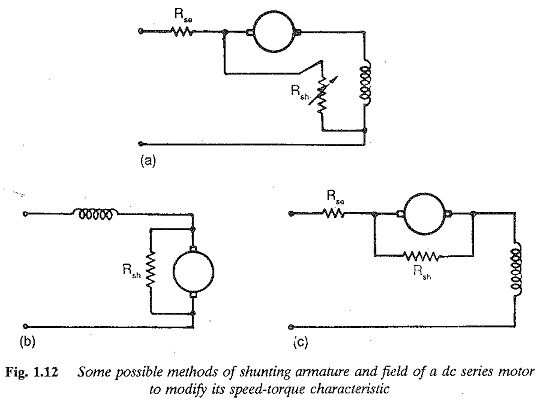A resistance connected across the armature and field provides a speed con­trol downward from its base speed (Fig. 1.12(a)). The voltage drop across the series resistance causes a speed drop and the torque developed at this speed is reduced by diverting the current through Rsh. In this connection the field and armature currents are the same. Hence it fails to provide a light load operation at low or medium speeds. However, the shift of the speed-torque characteristic towards the left is more than that achieved with simple conventional rheostatic control.

A better connection is shown in Fig. 1.12(b) where a resistance is shunted across the armature. Thus, only a part of the line current flows in the armature and the field current is maintained at the line value. By properly selecting the shunting resistance, a desirable speed-torque characteristic may be obtained. The higher field current stabilises the speed of lower values and the smaller armature current develops a lower torque. Very low speed operation is made possible by further connecting a resistance in series with the field. The shift of the characteristic towards the left is more due to Rs. Typical speed-torque curves are shown in Fig. 1.13. The characteristics show a greater hardness, being flat over a range of speeds. The method, however, is not economical due to losses in the resistances and is suitable for driving the load at low speeds for short intervals of time. A wide range of speeds below the base speed is not attainable with this method.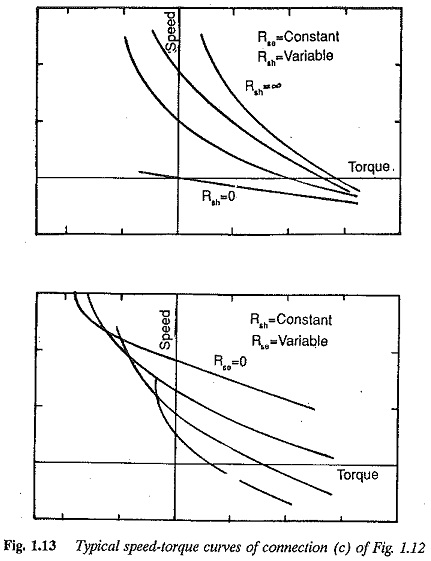When medium speeds are required, the shunting resistance may be discon7 nected. The motor then operates with series resistance Rs. When Rs is short circuited the motor runs on its natural characteristic. It is possible to operate the motor from no-load to full load at very low, medium and high speeds.

While lowering the load using a hoist, sometimes, the empty cage may have to be driven at low, medium or high speeds. High speed operation may be required for rapid lowering. A suitable connection for controlling the speed in such cases is shown in Fig. 1.14. The scheme is called the potentiometer lowering circuit. In this connection, the field of the motor is connected across the armature. A variable resistance Rse is connected in series with the motor. The armature of the dc motor gets its supply from the potential. divider formed by Rse and the combined resistance of the field and Rsh. The motor speed-torque characteristic can be derived from the following equations.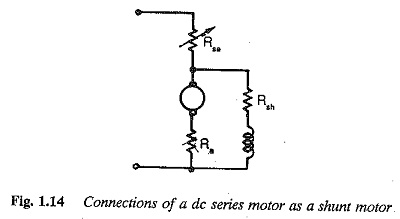The armature voltage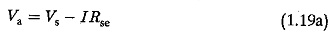The field current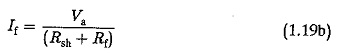The armature current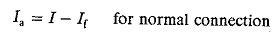The back emf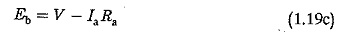The torque developed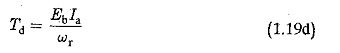The motor has a shunt characteristic. The derived speed-torque curves may be obtained by varying the resistance in series with the field. Reverse rotation is achieved by reversing the supply to the armature. A set of speed torque obtained by this connection is shown in Fig. 1.15, which indicates the possi­bility of operation in the fourth quadrant. The series field in this connection carries rated current and hence the limiting resistor in series with the field dissipates a considerable amount of energy. Typical speed-torque curves of a series motor in this application are shown in Fig. 1.16. The curves in the third quadrant show the operation of the hoist driving an empty cage at all speeds. The curves of the fourth quadrant show that heavy loads may be low­ered at these speeds. These may be obtained by the connection of Fig. 1.17. The speed-torque curves in the fourth quadrant may be obtained using the connection of Fig. 1.17(a).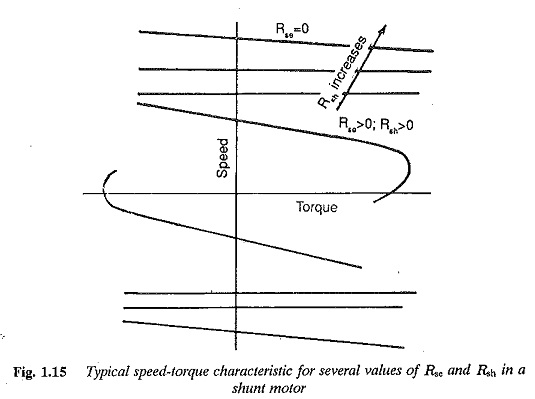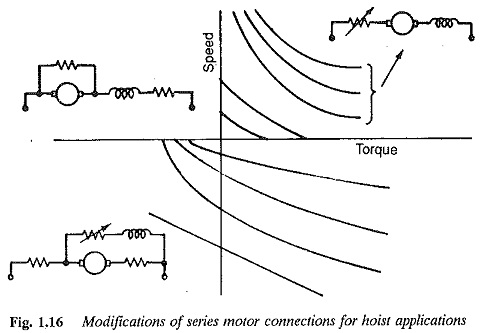Updated: September 30, 2017 — 9:40 pm